Win up to 100% scholarship on Aakash BYJU'S JEE/NEET courses with ABNAT Win up to 100% scholarship on Aakash BYJU'S JEE/NEET courses with ABNAT

# JEE Main 2022 June 27 – Shift 2 Physics Question Paper with Solutions

This page contains the JEE Main 2022 June 27 – Shift 2 Physics Question Paper with Solutions to guide students in their preparation, and ensure they pass the entrance exam. Students can get a detailed JEE main 2022 question paper with solutions that have been developed by our subject experts. By receiving only accurate responses to each question, students will learn and better understand the subject being covered. The JEE Main 2022 Physics question paper PDF is available below for students to read or download. As an alternative, students can work through the questions on their own, without consulting the answers. By doing this, students will improve their speed and accuracy, in addition to evaluating how prepared they are.

## JEE Main 2022 June 27th Physics Shift 2 Question Paper and Solutions

#### SECTION – A

Multiple Choice Questions: This section contains 20 multiple choice questions. Each question has 4 choices (1), (2), (3) and (4), out of which ONLY ONE is correct.

1. The SI unit of a physical quantity is pascal-second. The dimensional formula of this quantity will be :

(A) [ML–1T–1]

(B) [ML–1T–2]

(C) [ML2T–1]

(D) [M–1L3T0]

Sol. [pascal-second]

$$\begin{array}{l}=\frac{MLT^{-2}}{L^2}\times T\end{array}$$

= ML–1T–1

2. The distance of the Sun from Earth is 1.5 × 1011 m, and its angular diameter is (2000) s when observed from the earth. The diameter of the Sun will be :

(A) 2.45 × 1010 m

(B) 1.45 × 1010 m

(C) 1.45 × 109 m

(D) 0.14 × 109 m

Sol. Diameter = r × δ

$$\begin{array}{l}=1.5 \times 10^{11} \times \left ( 2000 \right ) \times \left ( \frac{1}{3600} \right )\times \left ( \frac{x}{180} \right )\end{array}$$

= 1.45 × 109 m

3. When a ball is dropped into a lake from a height 4.9 m above the water level, it hits the water with a velocity v and then sinks to the bottom with the constant velocity v. It reaches the bottom of the lake 4.0 s after it is dropped. The approximate depth of the lake is :

(A) 19.6 m

(B) 29.4 m

(C) 39.2 m

(D) 73.5 m

Sol.

$$\begin{array}{l}t_1=\sqrt{\frac{2h}{g}}\end{array}$$
$$\begin{array}{l}=\sqrt{\frac{2\times 4.9}{9.8}}= 1 \text{ s} \end{array}$$

Δt = 4 – 1 = 3 s,

$$\begin{array}{l}v=\sqrt{2gh}=\sqrt{2\times9.8\times4.9} = 9.8\ m/s \end{array}$$

∴ Depth = 9.8 × 3 = 29.4 m

4. One end of a massless spring of spring constant k and natural length l0 is fixed while the other end is connected to a small object of mass m lying on a frictionless table. The spring remains horizontal on the table. If the object is made to rotate at an angular velocity ω about an axis passing through fixed end, then the elongation of the spring will be :

$$\begin{array}{l}(A)\ \frac{k-m\omega^2l_0}{m\omega^2}\end{array}$$
$$\begin{array}{l}(B)\ \frac{m\omega^2l_0}{k+m\omega^2}\end{array}$$
$$\begin{array}{l}(C)\ \frac{m\omega^2l_0}{k-m\omega^2}\end{array}$$
$$\begin{array}{l}(D)\ \frac{k+m\omega^2l_0} {m\omega^2}\end{array}$$

Sol. mω2(l0 + x) = kx

mω2l0 = (kmω2) × x

$$\begin{array}{l}\Rightarrow x = \frac{m\omega^2l_0}{(k-m\omega^2)}\end{array}$$

5. A stone tied to a string of length L is whirled in a vertical circle with the other end of the string at the centre. At a certain instant of time, the stone is at its lowest position and has a speed u. The magnitude of change in its velocity, as it reaches a position where the string is horizontal, is

$$\begin{array}{l}\sqrt{x(u^2-gL)}.\end{array}$$
The value of x is

(A) 3

(B) 2

(C) 1

(D) 5

Sol.

$$\begin{array}{l}\overrightarrow{v}=\sqrt{u^2-2gL\hat{j}}\end{array}$$$$\begin{array}{l}\overrightarrow{u}= u\hat{i}\end{array}$$
$$\begin{array}{l}\therefore\left| \overrightarrow{v}-\overrightarrow{u}\right| = \sqrt{(u^2 -2gL) + u^2}\end{array}$$
$$\begin{array}{l}=\sqrt{2u^2-2gL}\end{array}$$

x = 2

6. Four spheres each of mass m form a square of side d (as shown in figure). A fifth sphere of mass M is situated at the centre of square. The total gravitational potential energy of the system is: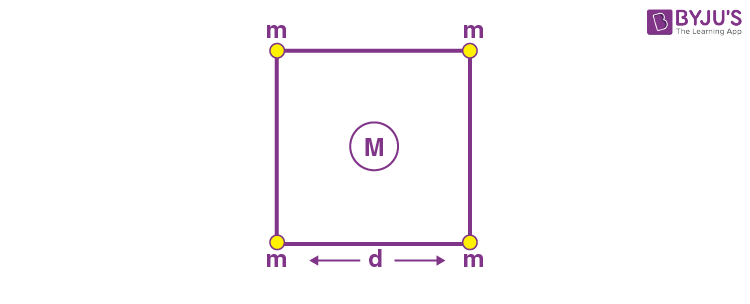(A)

$$\begin{array}{l}-\frac{Gm}{d} \left [ \left ( 4+\sqrt{2} \right )m + 4\sqrt{2}M \right ]\end{array}$$

(B)

$$\begin{array}{l}-\frac{Gm}{d} \left [ \left ( 4+\sqrt{2} \right )M + 4\sqrt{2}m \right ]\end{array}$$

(C)

$$\begin{array}{l}-\frac{Gm}{d} \left [ 3m^2+4\sqrt{2}M \right ]\end{array}$$

(D)

$$\begin{array}{l}-\frac{Gm}{d} \left [ 6m^2+4\sqrt{2}M \right ]\end{array}$$

Sol. Total gravitational potential energy

$$\begin{array}{l}=-\left\{ \frac{4GMm}{d/\sqrt{2}}+ \frac{4Gm^2}{d} + \frac{2Gm^2}{\sqrt{2}d}\right\}\end{array}$$
$$\begin{array}{l}=- \frac{Gm}{d}\left\{M4\sqrt{2}+(4+\sqrt{2})m \right\}\end{array}$$
$$\begin{array}{l}=- \frac{Gm}{d}\left\{4\sqrt{2}M +(4+\sqrt{2})m \right\}\end{array}$$

7. For a perfect gas, two pressures P1 and P2 are shown in the figure. The graph shows:(A) P1>P2

(B) P1<P2

(C) P1 = P2

(D) Insufficient data to draw any conclusion

Sol. As per ideal gas equation,

$$\begin{array}{l}V=\frac{nR}{P}T\end{array}$$

⇒Slope of V-T graph is inversely proportional to P.

As m2 > m1P1 > P2

8. According to kinetic theory of gases,

A. The motion of the gas molecules freezes at 0°C

B. The mean free path of gas molecules decreases if the density of molecules is increased.

C. The mean free path of gas molecules increases if temperature is increased keeping pressure constant.

D. Average kinetic energy per molecule per degree of freedom is

$$\begin{array}{l}\frac{3}{2}k_B T\end{array}$$
(for monoatomic gases).

Choose the most appropriate answer from the options given below:

(A) A and C only

(B) B and C only

(C) A and B only

(D) C and D only

Sol. According to kinetic theory of gases,

A. The motion of the gas molecules freezes at 0 K.

B. The mean free path decreases on increasing the number density of the molecules as

$$\begin{array}{l}\mu= \frac{1}{\sqrt{2}\pi n d^2} \Rightarrow \mu \propto \frac{1}{n}.\end{array}$$

C. The mean free path increases on increasing the volume. Now if temperature is increased by keeping the pressure constant the volume should increase that is mean free path increases.

D. K.E.avg per molecule per degree of freedom is

$$\begin{array}{l}\frac{1}{2}k_BT\end{array}$$

⇒ Option (B) and (C) only are correct.

9. A lead bullet penetrates into a solid object and melts. Assuming that 40% of its kinetic energy is used to heat it, the initial speed of bullet is:

(Given initial temperature of the bullet = 127°C),

Melting point of the bullet = 327°C,

Latent heat of fusion of lead = 2.5 × 104 J kg–1

Specific heat capacity of lead = 125 J/kg K)

(A) 125 ms–1

(B) 500ms–1

(C) 250ms–1

(D) 600ms–1

Sol.

$$\begin{array}{l}\frac{2}{5}\times\frac{1}{2}mv^2 = mL + ms\Delta T \end{array}$$
$$\begin{array}{l}\Rightarrow \frac{v^2}{5}= 2.5 \times 10^4 + 125 + 200\end{array}$$
$$\begin{array}{l}\Rightarrow \frac{v^2}{5}= 5 \times 10^4\end{array}$$

v = 500 m/s

10. The equation of a particle executing simple harmonic motion is given by

$$\begin{array}{l}x=sin \pi \left ( t + \frac{1}{3} \right )m.\end{array}$$
At t = 1 s, the speed of the particle will be

(A) 0 cm s–1

(B) 157cm s–1

(C) 272cm s–1

(D) 314cm s–1

Sol.

$$\begin{array}{l}x=sin \left ( \pi t + \frac{\pi}{3} \right )m\end{array}$$
$$\begin{array}{l}\Rightarrow \frac{dx}{dt} = \pi cos \left ( \pi t + \frac{\pi}{3} \right )\end{array}$$
$$\begin{array}{l}=\pi cos \left ( \pi + \frac{\pi}{3} \right )\end{array}$$
at t = 1 s

$$\begin{array}{l}= – \frac{\pi}{2}\text{m/s}\end{array}$$

or

$$\begin{array}{l}\left|\frac{dx}{dt} \right|= 157\ cm/s\end{array}$$

11. If a charge q is placed at the centre of a closed hemispherical non-conducting surface, the total flux passing through the flat surface would be:$$\begin{array}{l}(A)\ \frac{q}{ \varepsilon_0}\end{array}$$
$$\begin{array}{l}(B)\ \frac{q}{ 2\varepsilon_0}\end{array}$$
$$\begin{array}{l}(C)\ \frac{q}{ 4\varepsilon_0}\end{array}$$
$$\begin{array}{l}(D)\ \frac{q}{2\pi\varepsilon_0}\end{array}$$

Sol. Flux passing through flat surface = Flux passing through curved surface.

So

$$\begin{array}{l}\phi=\frac{q}{ 2\varepsilon_0} \end{array}$$

12. Three identical charged balls each of charge 2 C are suspended from a common point P by silk threads of 2 m each (as shown in figure). They form an equilateral triangle of side 1 m. The ratio of net force on a charged ball to the force between any two charged balls will be: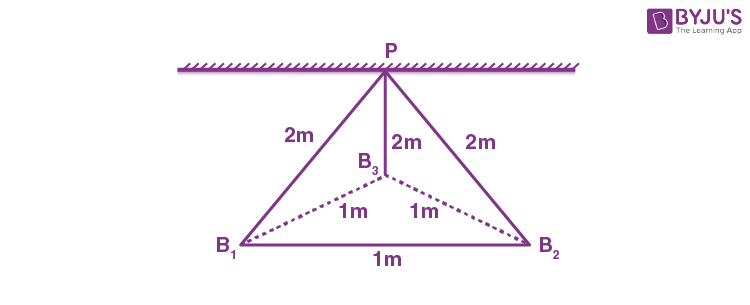(A) 1 : 1

(B) 1 : 4

(C) √3 : 2

(D) √3 : 1

Sol.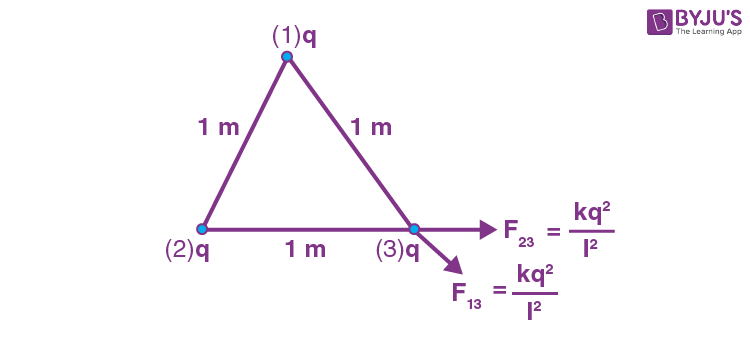Fnet on charge 3,

$$\begin{array}{l}F_1 = \frac{\sqrt{3}kq^2}{1^2}\end{array}$$

Force between any 2 charges

$$\begin{array}{l}F_2 = \frac{kq^2}{1^2}\end{array}$$

So,

$$\begin{array}{l}\frac{F_1}{F_2} = \sqrt{3}\end{array}$$

13. Two long parallel conductors S1 and S2 are separated by a distance 10 cm and carrying currents of 4 A and 2 A respectively. The conductors are placed along x-axis in XY plane. There is a point P located between the conductors (as shown in figure). A charge particle of 3π coulomb is passing through the point P with velocity

$$\begin{array}{l}\overrightarrow{v} = \left ( 2 \hat{i} + 3\hat{j} \right )\ m/s;\ \text{where}\ \hat{i}\ \text{and}\ \hat{j}\end{array}$$
represents unit vector along x & y axis respectively. The force acting on the charge particle is
$$\begin{array}{l}4\pi \times 10^{-5}\left ( -x\hat{i} + 2 \hat{j} \right )N\end{array}$$
. The value of x is: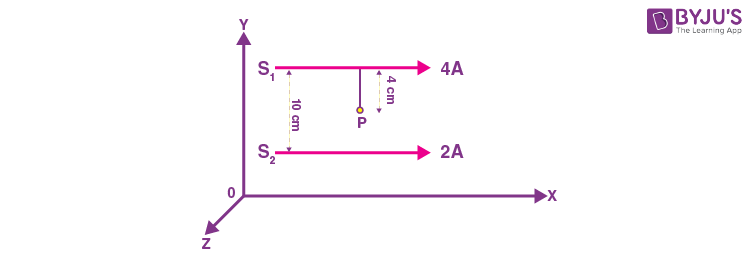(A) 2

(B) 1

(C) 3

(D) –3

Sol. Field at P is

$$\begin{array}{l}=\left ( \frac{\mu_0 \times i_1}{2\pi r_1} – \frac{\mu_0 i_2}{2\pi r_2} \right ) \left ( -\hat{k} \right )\end{array}$$
$$\begin{array}{l}=-\left ( \frac{\mu_0 4}{2\pi \times 0.04}- \frac{\mu_0 \times 2}{2\pi \times 0.06}\right ) \hat{k} = – \frac{\mu_0 \times 200}{6\pi} \hat{k}\end{array}$$

So, force

$$\begin{array}{l}\overrightarrow{F} = q\overrightarrow{v} \ \times \overrightarrow{B}\end{array}$$
$$\begin{array}{l}=3 \pi \left ( 2 \hat{i} + 3 \hat{j}\right ) \times \left ( -\left ( \frac{\mu_0 \ \times 200}{6 \pi} \right )\hat{k} \right )\end{array}$$
$$\begin{array}{l}=3\pi \left ( \frac{200 \mu_0}{3 \pi}\hat{j}-\frac{100 \mu_0}{\pi}\hat{i} \right )\end{array}$$
$$\begin{array}{l}=200\mu_0 \hat{j} – 300 \mu_0 \hat{i} \end{array}$$
$$\begin{array}{l}=4\pi \times 10^{-5}\left ( 2 \hat{j} – 3 \hat{i} \right )\end{array}$$

So, x = 3

14. If L, C and R are the self-inductance, capacitance and resistance, respectively, which of the following does not have the dimension of time?

(A) RC

$$\begin{array}{l}(B)\ \frac{L}{R}\end{array}$$
$$\begin{array}{l}(C)\ \sqrt{LC}\end{array}$$
$$\begin{array}{l}(D)\ \frac{L}{C}\end{array}$$

Sol.

$$\begin{array}{l}U=\frac{1}{2} Li^2 = \frac{1}{2}CV^2\end{array}$$

So,

$$\begin{array}{l}\left [ \frac{L}{C} \right ] = \frac{V^2}{i^2}=R^2\end{array}$$
is not the dimension of time.

15. Given below are two statements:

Statement I: A time varying electric field is a source of changing magnetic field and vice-versa. Thus a disturbance in electric or magnetic field creates EM waves.

Statement II: In a material medium, the EM wave travels with speed

$$\begin{array}{l}v=\frac{1}{\sqrt{\mu_0 \varepsilon_0 }} \end{array}$$

In the light of the above statements, choose the correct answer from the options given below.

(A) Both statement I and statement II are true

(B) Both statement I and statement II are false

(C) Statement I is correct but statement II is false

(D) Statement I is incorrect but statement II is true

Sol. In a material medium speed of light is given by:

$$\begin{array}{l}v=\frac{1}{\sqrt{\varepsilon_0 \varepsilon_r\mu_0 \mu_r }} \end{array}$$

So statement 2 is false.

16. A convex lens has power P. It is cut into two halves along its principal axis. Further one piece (out of the two halves) is cut into two halves perpendicular to the principal axis (as shown in figures). Choose the incorrect option for the reported pieces.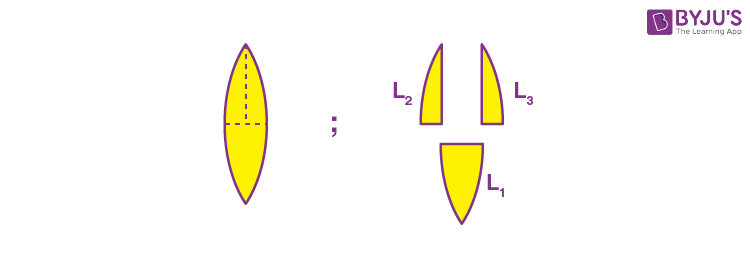$$\begin{array}{l}(A)\ \text{Power of}\ L_1 = \frac{P}{2}\end{array}$$
$$\begin{array}{l}(B)\ \text{Power of}\ L_2 = \frac{P}{2}\end{array}$$
$$\begin{array}{l}(C)\ \text{Power of}\ L_3 = \frac{P}{2}\end{array}$$

(D) Power of L1 = P

Sol. We know

$$\begin{array}{l}P = \frac{1}{f} = (\mu – 1) \left ( \frac{1}{R_1} – \frac{1}{R_2} \right )\end{array}$$
$$\begin{array}{l}L_1 : \frac{1}{f_1} = (\mu – 1) \left ( \frac{1}{R_1} – \frac{1}{R_2} \right ) = P_1 = (\mu – 1) \left ( \frac{2}{R} \right ) = P\end{array}$$
$$\begin{array}{l}L_2 : \frac{1}{f_2} = (\mu – 1) \left ( \frac{1}{R_1} \right ) = P_2 = \frac{\mu -1}{R}\end{array}$$
$$\begin{array}{l}L_3 : \frac{1}{f_3} = (\mu – 1) \left ( -\frac{1}{R_2} \right ) = P_3 = \frac{\mu -1}{R}\end{array}$$

17. If a wave gets refracted into a denser medium, then which of the following is true?

(A) Wavelength, speed and frequency decreases

(B) Wavelength increases, speed decreases and frequency remains constant

(C) Wavelength and speed decreases but frequency remains constant

(D) Wavelength, speed and frequency increases

Sol. Frequency is independent of medium. For denser medium, wavelength and speed both would decrease.

18. Given below are two statements:

Statement I: In hydrogen atom, the frequency of radiation emitted when an electron jumps from lower energy orbit (E1) to higher energy orbit (E2), is given as hf = E1E2.

Statement II: The jumping of electron from higher energy orbit (E2) to lower energy orbit (E1) is associated with frequency of radiation given as

$$\begin{array}{l}f=\frac{(E_2-E_1)}{h}\end{array}$$

This condition is Bohr’s frequency condition.

In the light of the above statements, choose the correct answer from the options given below:

(A) Both statement I and statement II are true

(B) Both statement I and statement II are false

(C) Statement I is correct but statement II is false

(D) Statement I is incorrect but statement II is true

Sol. Radiation is not emitted but absorbed when an electron jumps from low energy to high energy.

Also, E2E1 is the energy of photon

E2E1 = hf

$$\begin{array}{l}\Rightarrow f=\frac{E_2-E_1}{h}\end{array}$$

19. For a transistor to act as a switch, it must be operated in

(A) Active region

(B) Saturation state only

(C) Cut-off state only

(D) Saturation and cut-off state

Sol. A transistor acts as a switch when it is operated in saturation and cut-off state.

20. We do not transmit low frequency signal to long distance because-

(a) The size of the antenna should be comparable to signal wavelength which is unreal solution for a signal of longer wavelength

(b) Effective power radiated by a long wavelength baseband signal would be high

(c) We want to avoid mixing up signals transmitted by different transmitter simultaneously

(d) Low frequency signal can be sent to long distances by superimposing with a high frequency wave as well

Therefore, the most suitable option will be:

(A) All statements are true

(B) (a), (b) and (c) are true only

(C) (a), (c) and (d) are true only

(D) (b), (c) and (d) are true only

Sol. For longer wavelength, size of antenna would increase. Also, mixing of signals needs to be avoided.

Also, we can use modulation to send low frequency signal by superimposing them with high frequency signals.

#### SECTION – B

Numerical Value Type Questions: This section contains 10 questions. In Section B, attempt any five questions out of 10. The answer to each question is a NUMERICAL VALUE. For each question, enter the correct numerical value (in decimal notation, truncated/rounded-off to the second decimal place; e.g. 06.25, 07.00, –00.33, –00.30, 30.27, –27.30) using the mouse and the on-screen virtual numeric keypad in the place designated to enter the answer.

1. A mass of 10 kg is suspended vertically by a rope of length 5 m from the roof. A force of 30 N is applied at the middle point of rope in horizontal direction. The angle made by upper half of the rope with vertical is θ = tan–1 (x × 10–1). The value of x is _______.

(Given, g = 10 m/s2)

Sol.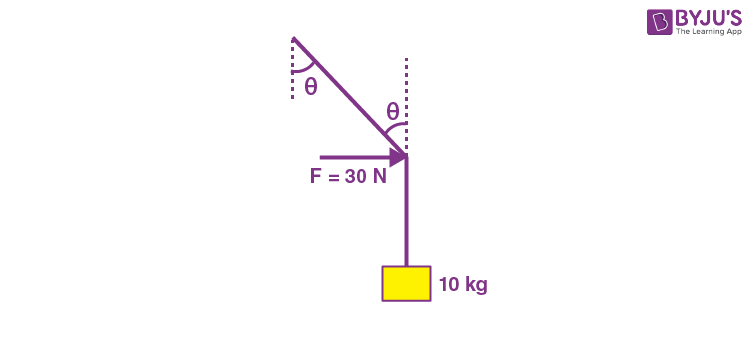T cosθ = mg

T cosθ = 100 N …(i)

T sinθ = 30 …(ii)

$$\begin{array}{l}\Rightarrow \frac{T sin \theta}{T cos \theta} = \frac{30}{100}\end{array}$$
$$\begin{array}{l}\Rightarrow tan ~\theta = \frac{3}{10}\end{array}$$

x = 3

2. A rolling wheel of 12 kg is on an inclined plane at position P and connected to a mass of 3 kg through a string of fixed length and pulley as shown in figure. Consider PR as friction free surface.

The velocity of centre of mass of the wheel when it reaches at the bottom Q of the inclined plane PQ will be

$$\begin{array}{l}\frac{1}{2} \sqrt{xgh}\ m/s.\end{array}$$
The value of x is _________.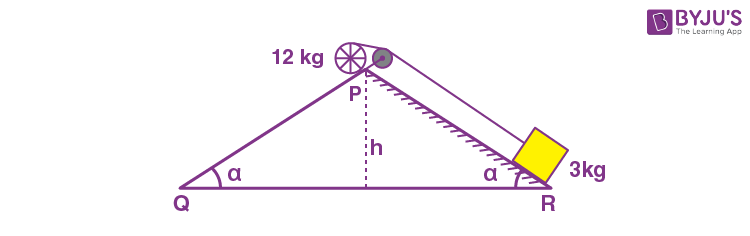Sol. For rolling wheel

[12g sinα – 3g sinα] × R = (2 × 12 R2 + 3R2) × (a/R)

$$\begin{array}{l}\Rightarrow \frac{9 g sin \alpha}{27}= a\end{array}$$
$$\begin{array}{l}\Rightarrow a = \frac{g~ sin~ \alpha}{3}\end{array}$$
$$\begin{array}{l}\therefore v = \sqrt{2 \times \frac{g~ sin~ \alpha}{3}\times \frac{h}{sin~ \alpha} } = \sqrt{\frac{2}{3}gh}\end{array}$$
$$\begin{array}{l}= \frac{1}{2} \ \times \sqrt{\frac{8}{3}gh}\end{array}$$
$$\begin{array}{l}\therefore x = \frac{8}{3} = 2.67\end{array}$$

3. A diatomic gas (γ = 1.4) does 400 J of work when it is expanded isobarically. The heat given to the gas in the process is ________ J.

Sol. W = nRΔT = 400 J

∴ ΔQ = nCPΔT

$$\begin{array}{l}= n \times \frac{7}{2}R \times \Delta T = \frac{7}{2} \times (400) = 1400\end{array}$$

4. A particle executes simple harmonic motion. Its amplitude is 8 cm and time period is 6 s. The time it will take to travel from its position of maximum displacement to the point corresponding to half of its amplitude, is _________ s.

Sol. A = 8 cm

T = 6 s

$$\begin{array}{l}A ~cos \left ( \frac{2 \pi t}{T} \right ) = \frac{A}{2}\end{array}$$
$$\begin{array}{l}\Rightarrow \frac{2\pi t}{T}= \frac{\pi}{3}\end{array}$$

or

$$\begin{array}{l}t = \frac{T}{6} = 1 ~\text{s} \end{array}$$

5. A parallel plate capacitor is made up of stair like structure with a plate area A of each stair and that is connected with a wire of length b, as shown in the figure. The capacitance of the arrangement is

$$\begin{array}{l}\frac{x}{15} \frac{\varepsilon _0 A}{b}\end{array}$$
, the value of x is ______?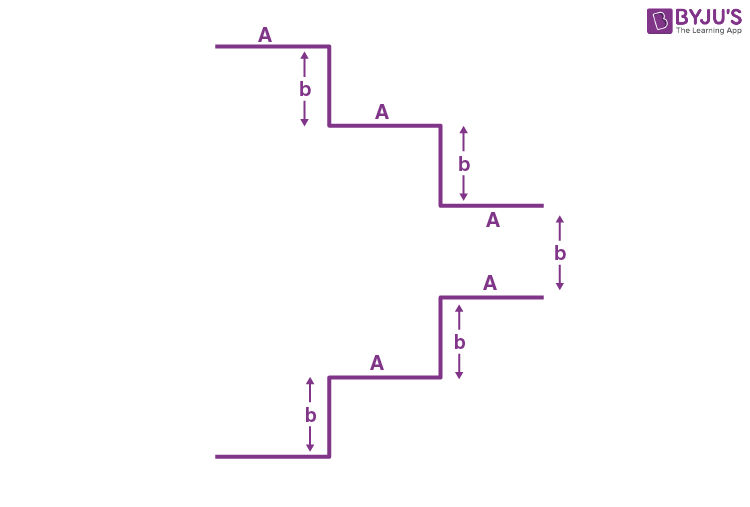Sol. The circuit is equivalent to 3 capacitors in parallel as shown.$$\begin{array}{l}C_{eq} = \frac{\varepsilon_0 A}{b} \left ( 1+ \frac{1}{3} + \frac{1}{5}\right )= \frac{23}{15} \frac{\varepsilon_0 A}{b} \end{array}$$

x = 23

6. The current density in a cylindrical wire of radius r = 4.0 mm is 1.0 x 106 A/m2. The current through the outer portion of the wire between radial distances r/2 and r is xπ A; where x is _________.

Sol. i = A × j

$$\begin{array}{l}= \pi \left ( R^2 – \frac{R^2}{4} \right )j\end{array}$$
$$\begin{array}{l}= \frac{3 \pi R^2}{4} \times j\end{array}$$
$$\begin{array}{l}= \frac{3 \pi \times (4 \times 10^{-3})^2} {4} \times 1.0 \times 10^6\end{array}$$

= 12 π

7. In the given circuit ‘a‘ is an arbitrary constant. The value of m for which the equivalent circuit resistance is minimum, will be

$$\begin{array}{l}\sqrt{\frac{x}{2}}.\end{array}$$
The value of x is________.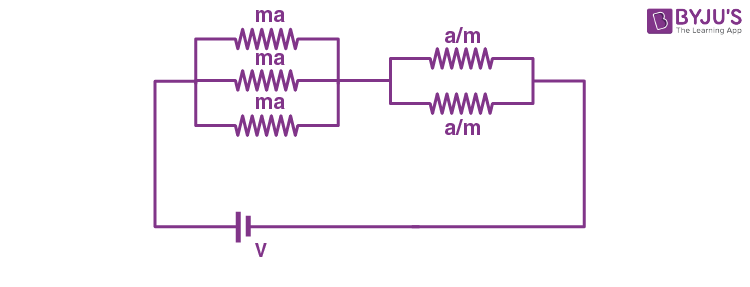Sol.

$$\begin{array}{l}R_{net}= \frac{ma}{3} + \frac{a}{2m}\end{array}$$
$$\begin{array}{l}=a\left [ \frac{m}{3} + \frac{1}{2m} – \frac{2}{\sqrt{6}} + \frac{2}{\sqrt{6}} \right ]\end{array}$$
$$\begin{array}{l}=a \left [ \left ( \sqrt{\frac{m}{3}}- \frac{1}{\sqrt{2m}} \right )^2 + \sqrt{\frac{2}{3}}\right ]\end{array}$$

This will be minimum when

$$\begin{array}{l}\sqrt{\frac{m}{3}} = \frac{1}{\sqrt{2m}}\end{array}$$

or

$$\begin{array}{l} m = \sqrt{\frac{3}{2}}\end{array}$$
so x = 3

8. A deuteron and a proton moving with equal kinetic energy enter into a uniform magnetic field at right angle to the field. If rd and rp are the radii of their circular paths respectively, then the ratio

$$\begin{array}{l}\frac{r_d}{r_p}\ \text{will be}\ \sqrt{x} : 1\end{array}$$
where x is __________.

Sol.

$$\begin{array}{l}R = \frac{\sqrt{2mK}}{qB}\end{array}$$

So

$$\begin{array}{l}\frac{r_d}{r_p} = \frac{\sqrt{m_d}/q_d}{\sqrt{m_p/q_p}}\end{array}$$
$$\begin{array}{l}=\sqrt{2}\end{array}$$

So x = 2

9. A metallic rod of length 20 cm is placed in North-South direction and is moved at a constant speed of 20 m/s towards East. The horizontal component of the Earth’s magnetic field at that place is 4 × 10–3 T and the angle of dip is 45º. The emf induced in the rod is __________mV.

Sol. E = Blv

$$\begin{array}{l} =4\times 10^{-3}\times \frac{20}{100}\times 20\ \text{Volts}\end{array}$$

= 16 mV

10. The cut-off voltage of the diodes (shown in figure) in forward bias is 0.6 V. The current through the resistor of 40 Ω is ____ mA.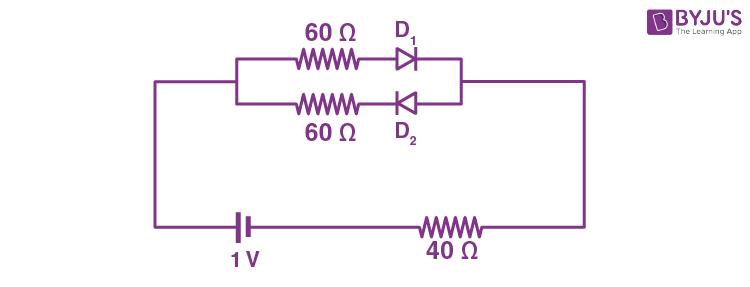Sol. D1 : conducting

D2 : open circuit

$$\begin{array}{l}\Rightarrow i = \frac{1-0.6}{60+40}A\end{array}$$
$$\begin{array}{l}=\frac{0.4}{100} \textup{A}\end{array}$$

i = 4 mA

### Download PDF of JEE Main 2022 June 27 Shift 2 Physics Paper & Solutions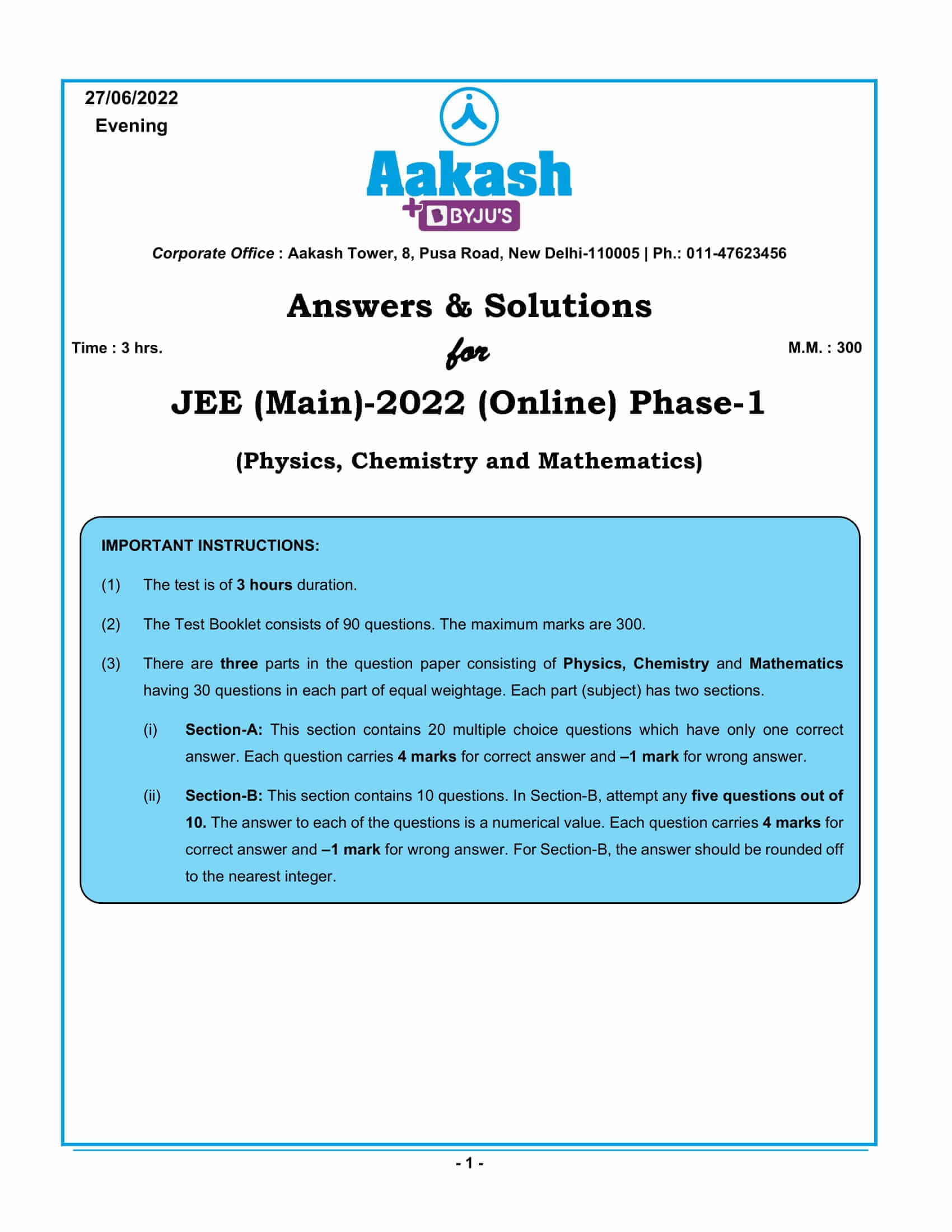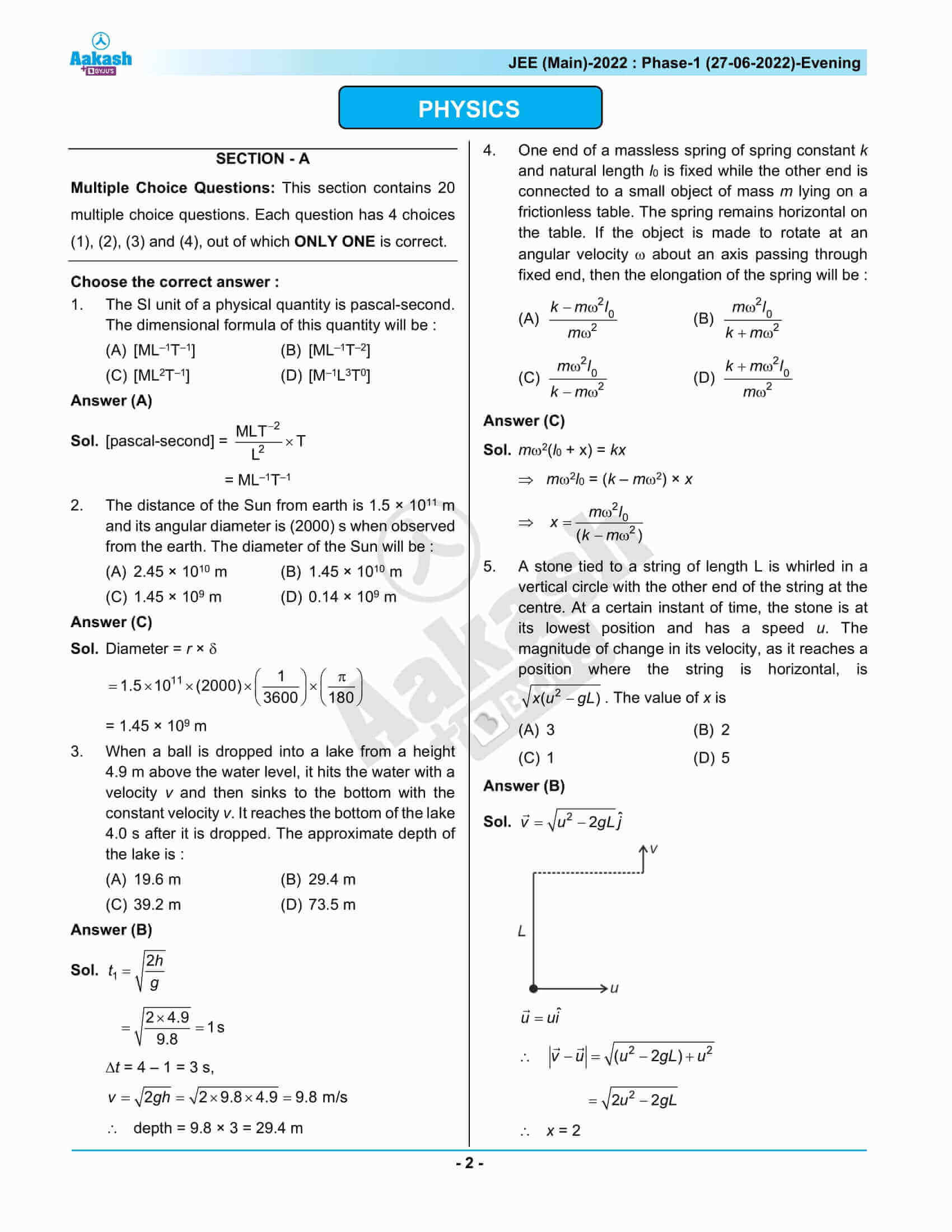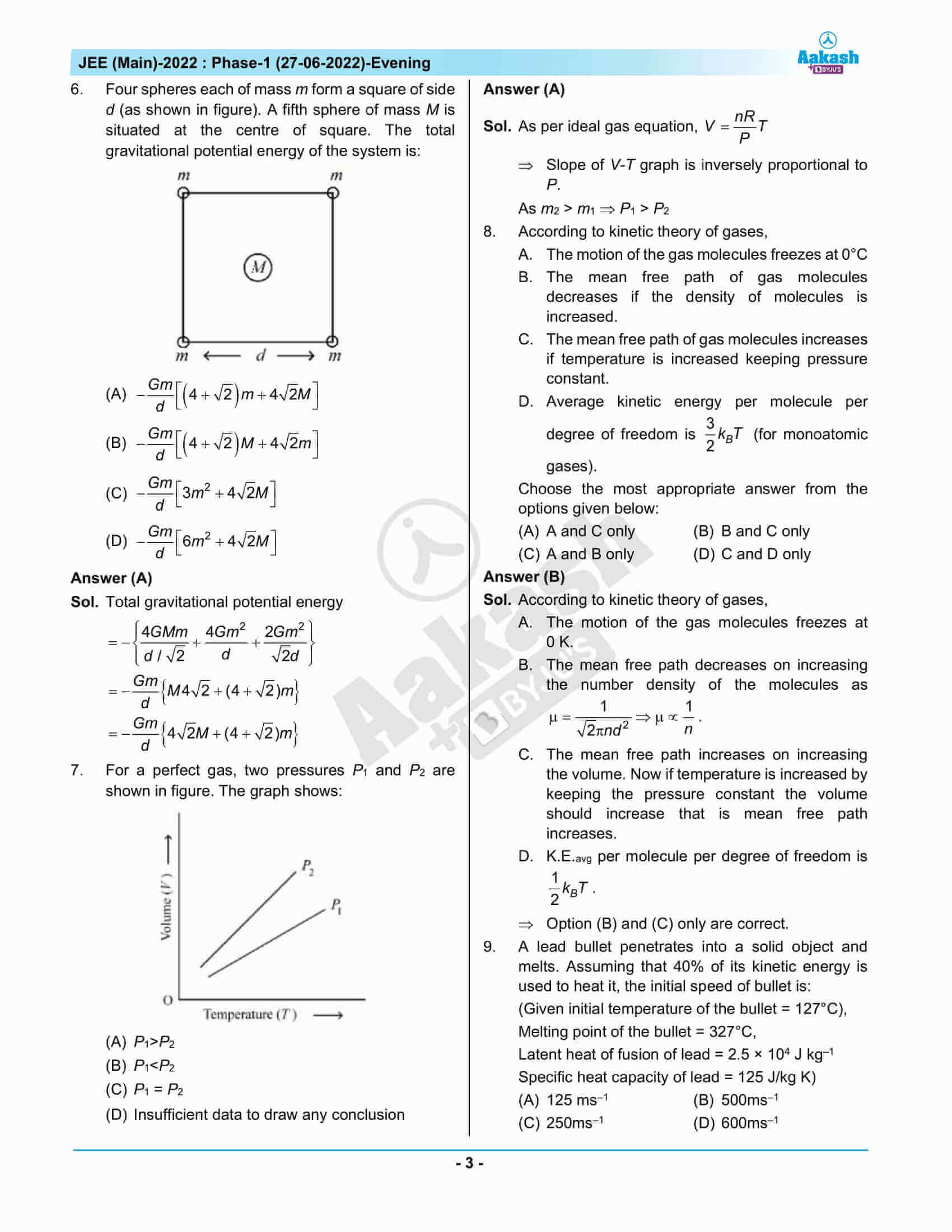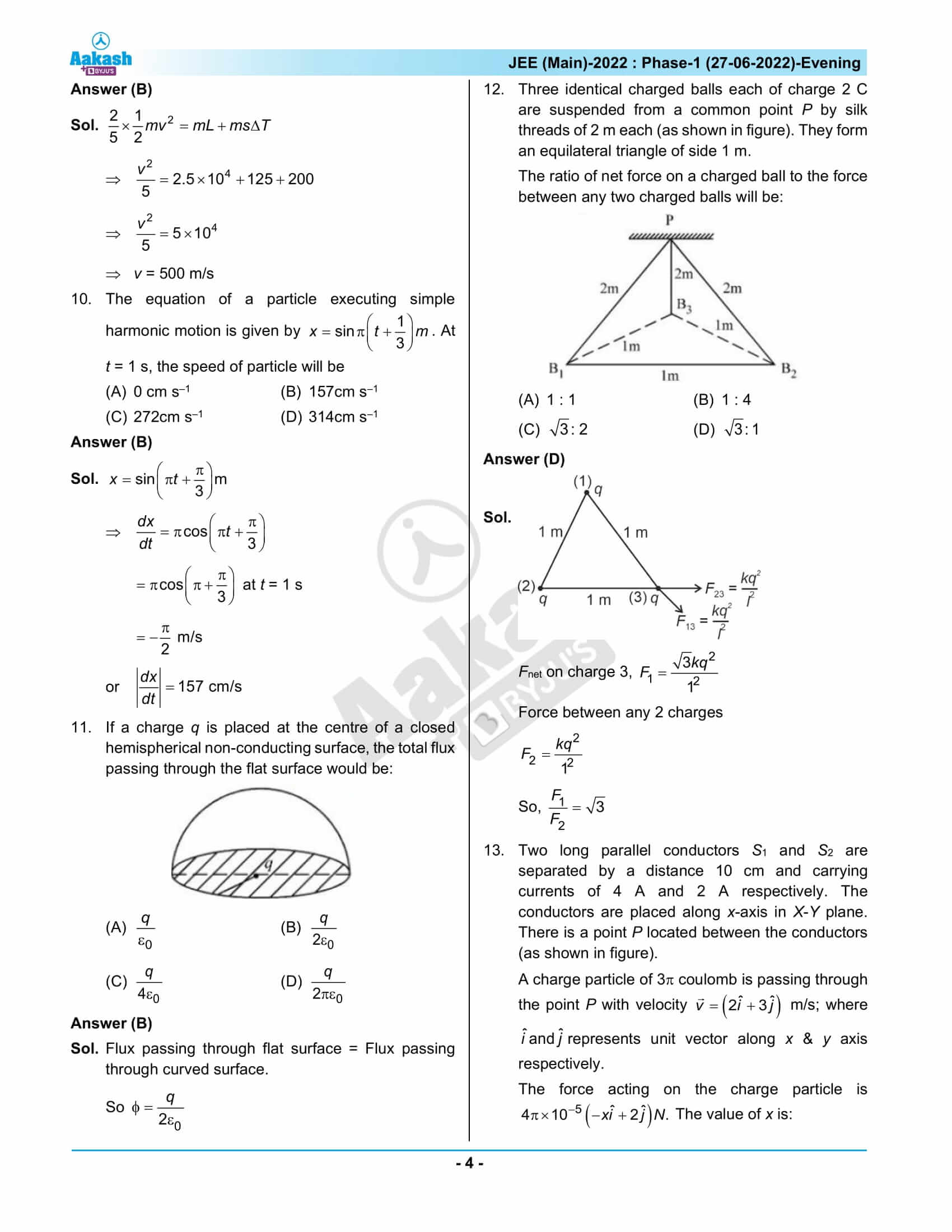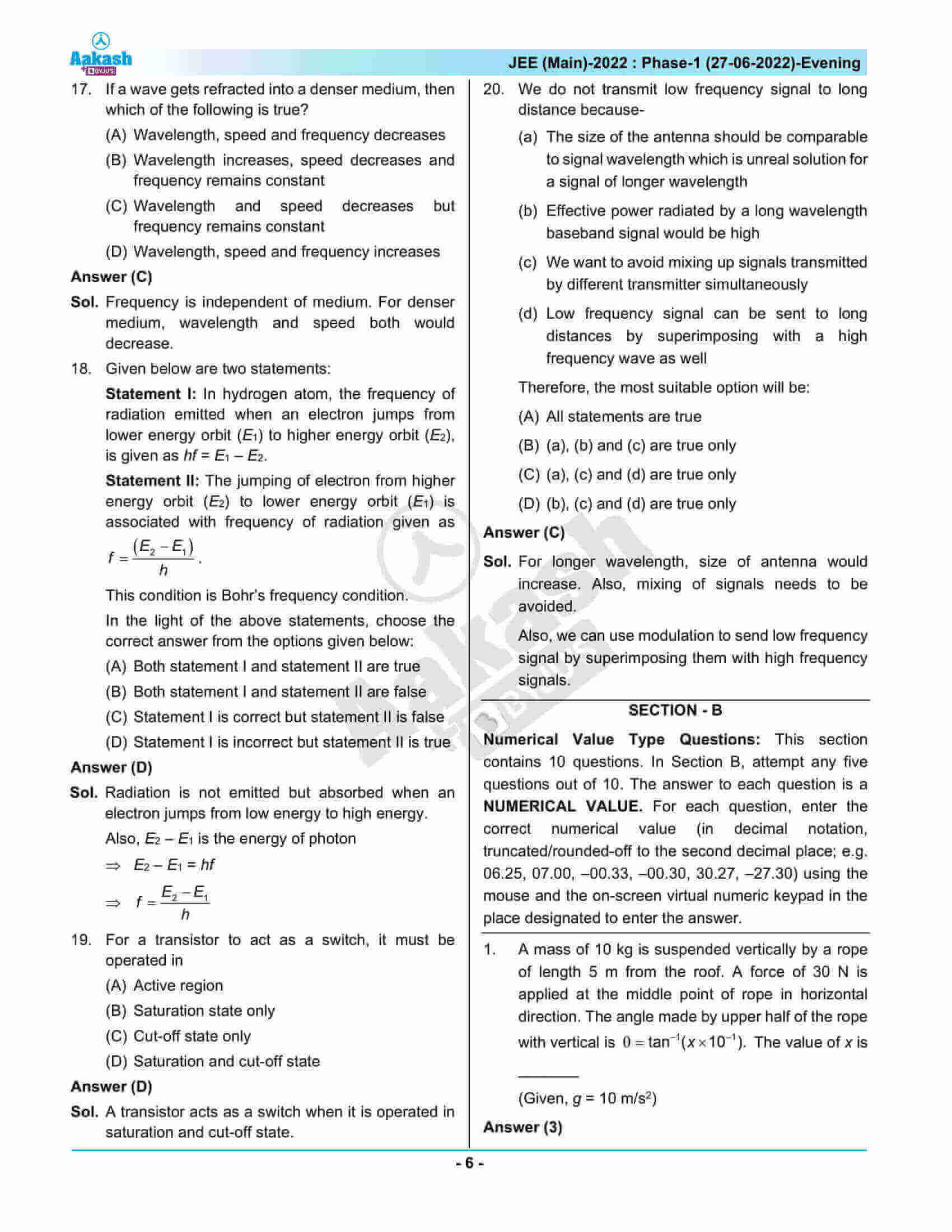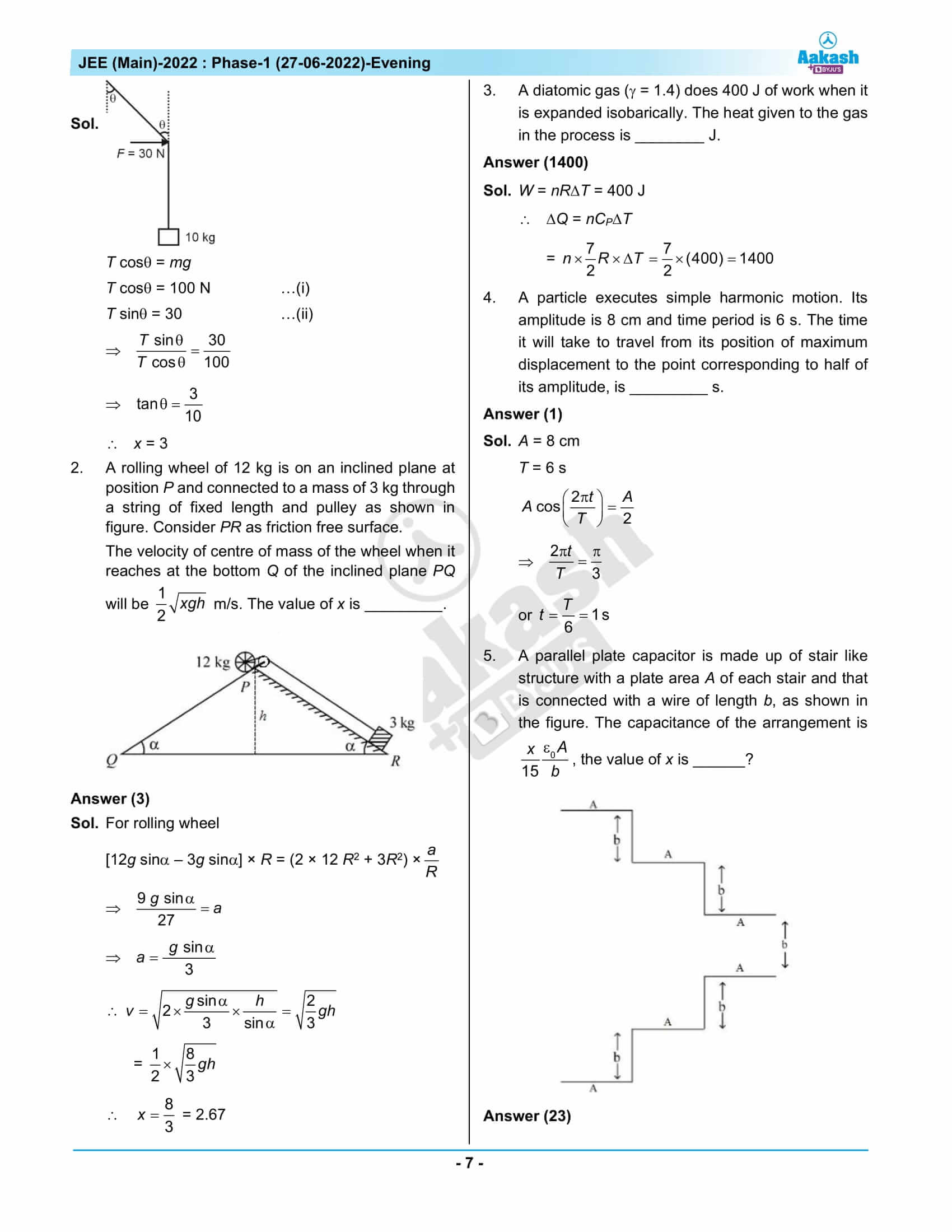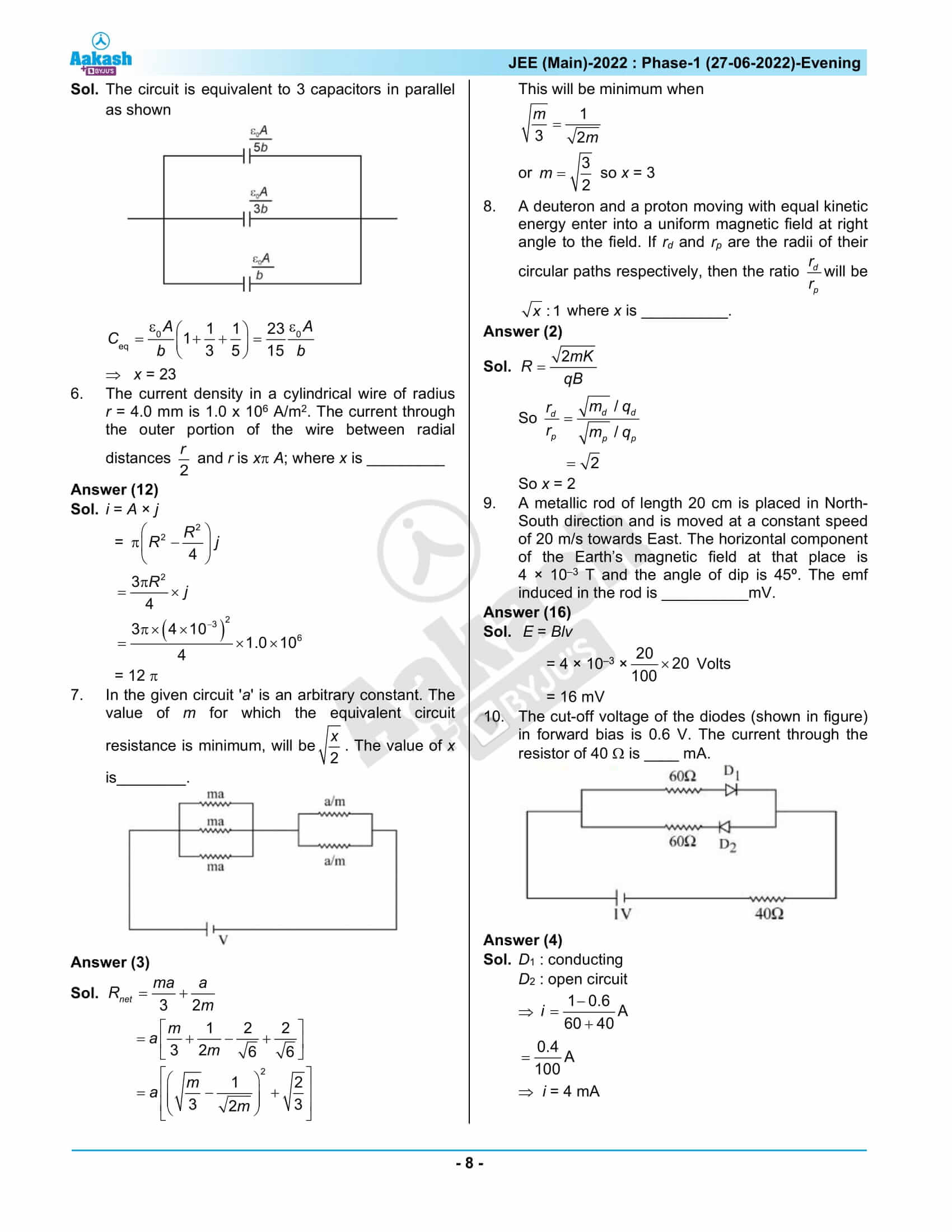## JEE Main 2022 Question Paper with Solutions June 27 Shift 2

### JEE Main 2022 June 27 Shift 2 Question Paper – Physics Solutions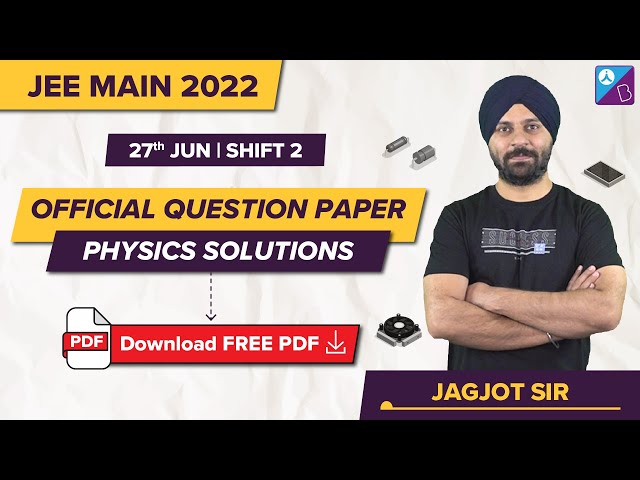### JEE Main 2022 June 27 Shift 2 Question Paper – Chemistry Solutions### JEE Main 2022 June 27 Shift 2 Question Paper – Maths Solutions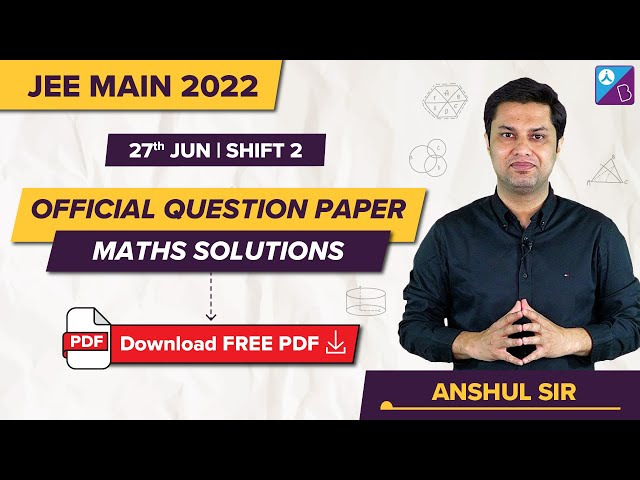## Frequently Asked Questions – FAQs

Q1

### What were the chapters included from Class 12 Physics in the afternoon shift question paper of JEE Main conducted on June 27, 2022?

The topics included from the Class 12 Physics syllabus were
Electrostatic Potential and Capacitance
Moving Charges and Magnetism
Ray Optics and Optical Instruments
Electric Charges and Fields
Semiconductor Electronics: Materials, Devices and Simple Circuits
Current Electricity
Atoms
Magnetism and Matter
Communication Systems

Q2

### Mention a few Physics topics from the Class 11 syllabus from which questions were asked in JEE Main 2022 June 27 Shift 2?

Few of the Physics topics from which questions were included in JEE Main 2022 June 27 Shift 2 were:
Thermodynamic Processes
GaussS Law
Junction Transistor (Transistor as a switch)
Gravitational Potential Energy
Vector Algebra
Simple Harmonic Motion
Application of Laws of Motion
Kinetic Theory of An Ideal Gas
Equilibrium of forces

Q3

### What was the difficulty level of the Physics questions asked in JEE Main 2022 June 27 Shift 2?

The Physics question paper can be rated 1.67 on a scale of 1 to 3.

Q4

### Which subject was the easiest in JEE Main 2022 June 27 Shift 2 question paper?

In comparison to Mathematics and Chemistry, Physics was easy.

Q5

### Can the Physics questions asked in EE Main 2022 June 27 Shift 2 be categorized as a simple, medium, or difficult?

From the list of memory-based questions, it can be seen that there were 10 easy questions, 12 medium-type questions and 2 difficult questions.